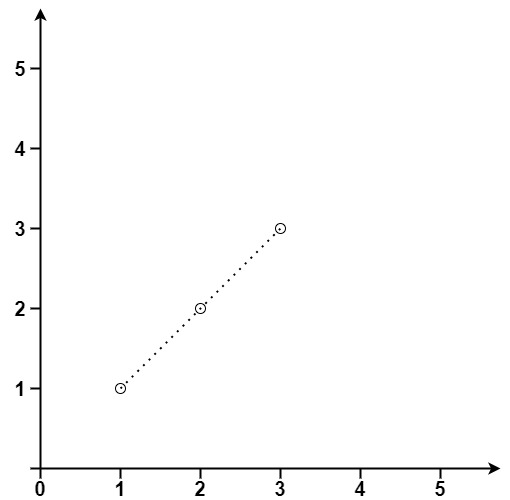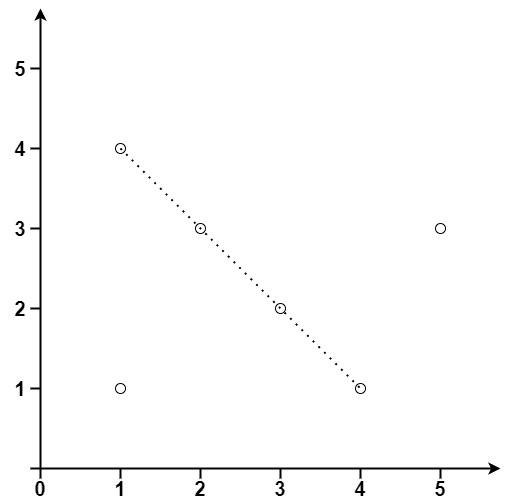Points in Straight Line
Easy Accuracy: 26.78% Submissions: 5622 Points: 2

Given two arrays X[] and Y[] of points where (Xi, Yi) represents a point on the X-Y plane. Your task is to complete the function maxPoints which returns an integer denoting the maximum number of points that lie on the same straight line.

Example 1:

Input:
X[] = {1, 2, 3}
Y[] = {1, 2, 3}
Output: 3
Explanation:
The points are (1,1), (2,2) and (3,3).Example 2:

Input:
X[] = {1, 3, 5, 4, 2, 1}
Y[] = {1, 2, 3, 1, 3, 4}
Output: 4
Explanation:
The points are-
(1,1),(3,2),(5,3),(4,1),(2,3),(1,4)You don't need to read input or print anything. Your task is to complete the function maxPoints() which takes two lists of coordinates as input and returns the maximum number of points that lies on the same line.

Expected Time Complexity: O(N2)
Expected Auxiliary Space: O(N)

Constraints:

1 <= N <= 300
-104 <= xi, yi <= 104

We are replacing the old Disqus forum with the new Discussions section given below.

### Editorial

We strongly recommend solving this problem on your own before viewing its editorial. Do you still want to view the editorial?

#### My Submissions: